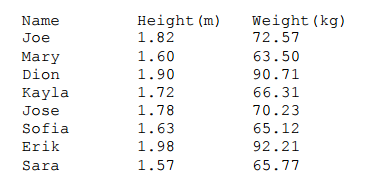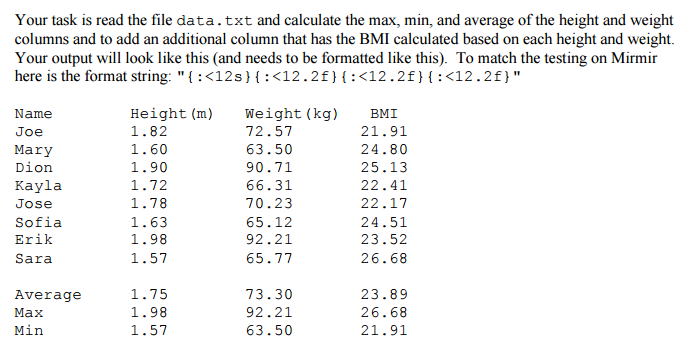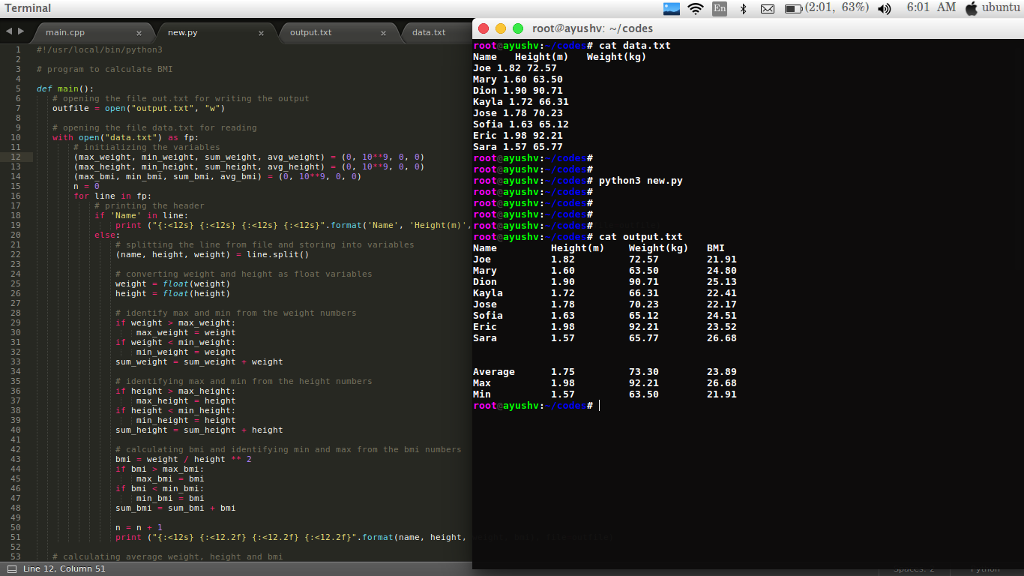# Answered! In python 3, how do I upload a txt file and manipulate it so that the file gives an average, max, and min and how do I…

In python 3, how do I upload a txt file and manipulate it so that the file gives an average, max, and min and how do I add a new column to the txt file? The txt file is below and is called “data” and gives the name, height, and weight. How do I add a new column for BMI which is weight/(height^2) and find the average, max, and min for the columns? The first image is what the txt file gives and the second image is what the python code is suppose to give and look like.Don't use plagiarized sources. Get Your Custom Essay on
Answered! In python 3, how do I upload a txt file and manipulate it so that the file gives an average, max, and min and how do I…
GET AN ESSAY WRITTEN FOR YOU FROM AS LOW AS \$13/PAGE#!/usr/local/bin/python3

# program to calculate BMI

def main():
# opening the file out.txt for writing the output
outfile = open(“output.txt”, “w”)

# opening the file data.txt for reading
with open(“data.txt”) as fp:
# initializing the variables
(max_weight, min_weight, sum_weight, avg_weight) = (0, 10**9, 0, 0)
(max_height, min_height, sum_height, avg_height) = (0, 10**9, 0, 0)
(max_bmi, min_bmi, sum_bmi, avg_bmi) = (0, 10**9, 0, 0)
n = 0
for line in fp:
if ‘Name’ in line:
print (“{:<12s} {:<12s} {:<12s} {:<12s}”.format(‘Name’, ‘Height(m)’, ‘Weight(kg)’, ‘BMI’), file=outfile)
else:
# splitting the line from file and storing into variables
(name, height, weight) = line.split()

# converting weight and height as float variables
weight = float(weight)
height = float(height)

# identify max and min from the weight numbers
if weight > max_weight:
max_weight = weight
if weight < min_weight:
min_weight = weight
sum_weight = sum_weight + weight

# identifying max and min from the height numbers
if height > max_height:
max_height = height
if height < min_height:
min_height = height
sum_height = sum_height + height

# calculating bmi and identifying min and max from the bmi numbers
bmi = weight / height ** 2
if bmi > max_bmi:
max_bmi = bmi
if bmi < min_bmi:
min_bmi = bmi
sum_bmi = sum_bmi + bmi

n = n + 1
print (“{:<12s} {:<12.2f} {:<12.2f} {:<12.2f}”.format(name, height, weight, bmi), file=outfile)

# calculating average weight, height and bmi
avg_weight = sum_weight / n
avg_height = sum_height / n
avg_bmi = sum_bmi / n
print (“n”, file=outfile)

# printing average, max, min statistics
print (“{:<12s} {:<12.2f} {:<12.2f} {:<12.2f}”.format(‘Average’, avg_height, avg_weight, avg_bmi), file=outfile)
print (“{:<12s} {:<12.2f} {:<12.2f} {:<12.2f}”.format(‘Max’, max_height, max_weight, max_bmi), file=outfile)
print (“{:<12s} {:<12.2f} {:<12.2f} {:<12.2f}”.format(‘Min’, min_height, min_weight, min_bmi), file=outfile)

fp.close()
outfile.close()

if __name__==’__main__’:
main()